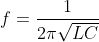What is the frequency of LC oscillator?

An LC circuit is used to either generate signals at a particular frequency or select a particular signal from a more complex signal. LC circuits are utilized in circuits such as oscillators, filters and tuners.

An LC circuit consists of two main components: the inductor (L) and the capacitor (C). The capacitors and inductors in the circuit can either be connected in parallel or series. There exists a resonant frequency in the circuit.

The resonant frequency is represented by the following equation:where f is the resonant frequency in hertz (Hz), L is the inductance in henries (H), C is the capacitance in farads F.(4)(1)